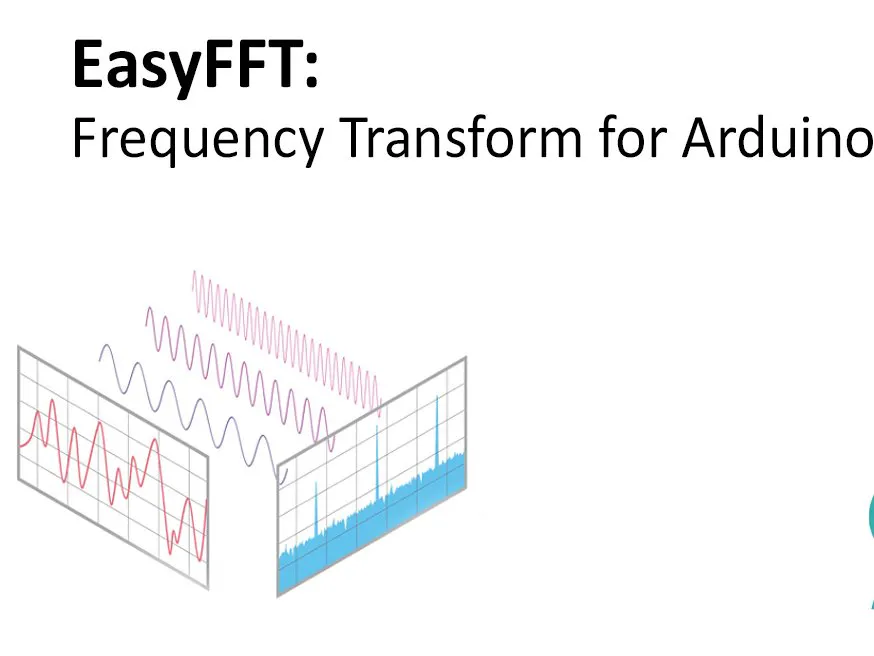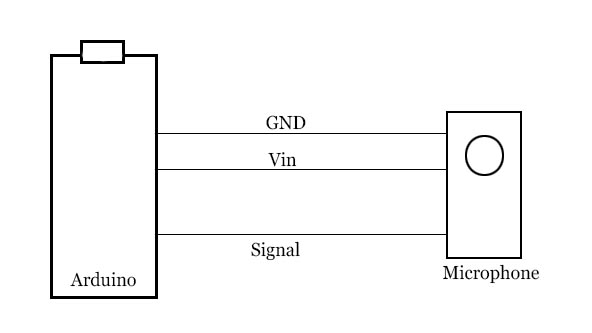Project tutorial# EasyFFT: Fast Fourier Transform (FFT) for Arduino © GPL3+

This code performs FFT with good accuracy.

• 44,502 views
• 23 respects

## Apps and online services

Measurement of frequency from the captured signal can be a difficult task, especially on Arduino as it has lower computational power. There are methods available to capture zero-crossing where the frequency is captured by checked how many times the signal crosses zero lines within the given time. Such a method may not work when the signal is a combination of various frequencies.

This is somehow difficult to code if you are not from such a background. But being a tinkerer this code may be highly useful for various projects related to music, signal analysis. The motive of this project was to prepare a code that is easy to implement on Arduino without getting into the background of it.

This project does not explain the working of FFT but explains the application of the FFT function. The same process is also explained in the attached video.

If you are only interested in the application of code and not into an explanation of it. You may skip directly to step no 3.

If you need to perform FFT with high speed (3x) with small compromise in accuracy (around 5%) refer my another article on ApproxFFT.

https://create.arduino.cc/projecthub/abhilashpatel121/approxfft-fastest-fft-function-for-arduino-fd4917?ref=user&ref_id=1593632&offset=0

### Step 1: Fast Fourier Transform

To make the computation of DFT faster FFT algorithm was developed by James Cooley and John Tukey. This algorithm is also considered as one of the most important algorithms of the 20th century. It divides a signal into an odd and even sequenced part which makes a number of required calculations lower. By using it total required complex multiplication can be reduced to NlogN. which is a significant improvement. Typical DFT takes N*N complex multiplication for results, while FFT only takes N*logN. this is a significant advantage when the sample numbers are high.

You may refer below references that I referred to while writing the code for a detailed understanding of the mathematics behind FFT:

### Step 2: Explanation of Code

1. Fast sine and Cosine:

Calculation FFT takes the value of various sine and cosine multiple times. The inbuilt function of Arduino is not fast enough and takes a good amount of time to provide the required value. Which makes code significantly slower (doubles time for 64 samples). To counter this issue value of sine for 0 to 90 degrees is stored as multiple of 255. Doing so will eliminate the need of using storing numbers as float and we can store it as byte which takes 1/4th space on Arduino. The sine_data[ ] needs to paste at top of code to declare it as a global variable.

Apart from sine_data, an array called f_peaks[] declared as a global variable. After every run of FFT function this array updates. Where f_peaks is the most dominant frequency and further values in descending order.

``````byte sine_data = {
0,
4,    9,    13,   18,   22,   27,   31,   35,   40,   44,
49,   53,   57,   62,   66,   70,   75,   79,   83,   87,
91,   96,   100,  104,  108,  112,  116,  120,  124,  127,
131,  135,  139,  143,  146,  150,  153,  157,  160,  164,
167,  171,  174,  177,  180,  183,  186,  189,  192,  195,
198,  201,  204,  206,  209,  211,  214,  216,  219,  221,
223,  225,  227,  229,  231,  233,  235,  236,  238,  240,
241,  243,  244,  245,  246,  247,  248,  249,  250,  251,
252,  253,  253,  254,  254,  254,  255,  255,  255,  255
};
float f_peaks;
``````

As we have stored value of sine for 0 to 90 degrees any value of sine or cosine can be calculated. Below function the first round of the number to zero decimal point and return value from stored data. this method needs only one floating division. This can be further reduced by directly storing sine values (not 255 multiple). but that eats up high memory on Arduino.

Using the above procedure reduces accuracy but improves speed. For 64 points, it gives the advantage of 8ms and for 128 points it gives an advantage of 20ms.

### Step 3: Explanation of Code: FFT Function

FFT can only be performed for the sample size of 2, 4, 8, 16, 32, 64 and so on. if the value is not 2^n, than it will take the lower side of value. For example, if we choose the sample size of 70 then it will only consider the first 64 samples and omit rest.

It is always recommended to have a sample size of 2^n. which can be:

2, 4, 8, 16, 32, 64, 128, 256, 512, 1024, 2048,...

Two floats out_r and out_im will take a high amount of memory. for Arduino nano won't work for samples higher than 128 (and in some cases 128) due to lack of available memory.

``````unsigned int data={1,2,4,8,16,32,64,128,256,512,1024,2048};
int a,c1,f,o,x;
a=N;

for(int i=0;i<12;i++)                 //calculating the levels
{ if(data[i]<=a){o=i;} }

int in_ps[data[o]]={};     //input for sequencing
float out_r[data[o]]={};   //real part of transform
float out_im[data[o]]={};  //imaginory part of transform
``````

Further flow is as follow:

1. Code generates a bit reversed the order for the given sample size (details on bit reversing on references: step 2)

2. Input data ordered as per generated order,

3. FFT performed

4. The amplitude of the complex number calculated,

5. Peaks are detected and ordered in descending order

6. results can be accessed from f_peaks[].

[to access other data (apart from peak frequency) code should be modified, so that local variable can be copied to some predefined global variable]

### Step 4: Testing the Code

A sample triangle wave is given as input. for this wave sampling frequency is 10 Hz and the frequency of wave itself is 1.25 Hz.

As can be shown from the raw output, value is matching with the FFT calculated by Scilab. however, these values are not exactly the same as we low accuracy but faster sine wave.

In output frequency array frequency are 1.25 and 3.75. it is not necessary to get the exact value every time. typically these numbers are called frequency bins. so output value might be anywhere within specified bins.

Speed:

for Arduino nano it takes:

• 16 Points : 4ms
• 32 Points : 10ms
• 64 Points : 26ms
• 128 Points : 53ms

### Step 5: Conclusion

This FFT code can be used in real-time applications. As it takes around 30 ms to complete the calculation. However, its resolution limited by a number of samples. The number of the sample is limited by Arduino memory. By using Arduino Mega or other higher performance board accuracy can be improved.

If you have any queries, suggestions, or corrections feel free to comment.

## Code

##### EasyFFTArduino
This code performs FFT and updates the F_peasks array with top 5 most dominent frequencies.
```/*
//Example data:
int data={14, 30, 35, 34, 34, 40, 46, 45, 30, 4,  -26,  -48,  -55,  -49,  -37,
-28,  -24,  -22,  -13,  6,  32, 55, 65, 57, 38, 17, 1,  -6, -11,  -19,  -34,
-51,  -61,  -56,  -35,  -7, 18, 32, 35, 34, 35, 41, 46, 43, 26, -2, -31,  -50,
-55,  -47,  -35,  -27,  -24,  -21,  -10,  11, 37, 58, 64, 55, 34, 13, -1, -7};
*/

//---------------------------------------------------------------------------//
byte sine_data =
{
0,
4,    9,    13,   18,   22,   27,   31,   35,   40,   44,
49,   53,   57,   62,   66,   70,   75,   79,   83,   87,
91,   96,   100,  104,  108,  112,  116,  120,  124,  127,
131,  135,  139,  143,  146,  150,  153,  157,  160,  164,
167,  171,  174,  177,  180,  183,  186,  189,  192,  195,       //Paste this at top of program
198,  201,  204,  206,  209,  211,  214,  216,  219,  221,
223,  225,  227,  229,  231,  233,  235,  236,  238,  240,
241,  243,  244,  245,  246,  247,  248,  249,  250,  251,
252,  253,  253,  254,  254,  254,  255,  255,  255,  255
};
float f_peaks; // top 5 frequencies peaks in descending order
//---------------------------------------------------------------------------//

void setup()
{
Serial.begin(250000);
}

void loop() {

/*
//example
FFT(data,64,100);        //to get top five value of frequencies of X having 64 sample at 100Hz sampling
Serial.println(f_peaks);
Serial.println(f_peaks);
delay(99999);
*/

/*
after ruing above FFT(), frequencies available at f_peaks,f_peaks,f_peaks,f_peaks,f_peaks,
*/
}

//-----------------------------FFT Function----------------------------------------------//

float FFT(int in[],int N,float Frequency)
{
/*
Code to perform FFT on arduino,
setup:
paste sine_data  at top of program [global variable], paste FFT function at end of program
Term:
1. in[]     : Data array,
2. N        : Number of sample (recommended sample size 2,4,8,16,32,64,128...)
3. Frequency: sampling frequency required as input (Hz)

If sample size is not in power of 2 it will be clipped to lower side of number.
i.e, for 150 number of samples, code will consider first 128 sample, remaining sample  will be omitted.
For Arduino nano, FFT of more than 128 sample not possible due to mamory limitation (64 recomended)
For higher Number of sample may arise Mamory related issue,
Code by ABHILASH
Contact: abhilashpatel121@gmail.com
Documentation:https://www.instructables.com/member/abhilash_patel/instructables/
2/3/2021: change data type of N from float to int for >=256 samples
*/

unsigned int data={1,2,4,8,16,32,64,128,256,512,1024,2048};
int a,c1,f,o,x;
a=N;

for(int i=0;i<12;i++)                 //calculating the levels
{ if(data[i]<=a){o=i;} }

int in_ps[data[o]]={};     //input for sequencing
float out_r[data[o]]={};   //real part of transform
float out_im[data[o]]={};  //imaginory part of transform

x=0;
for(int b=0;b<o;b++)                     // bit reversal
{
c1=data[b];
f=data[o]/(c1+c1);
for(int j=0;j<c1;j++)
{
x=x+1;
in_ps[x]=in_ps[j]+f;
}
}

for(int i=0;i<data[o];i++)            // update input array as per bit reverse order
{
if(in_ps[i]<a)
{out_r[i]=in[in_ps[i]];}
if(in_ps[i]>a)
{out_r[i]=in[in_ps[i]-a];}
}

int i10,i11,n1;
float e,c,s,tr,ti;

for(int i=0;i<o;i++)                                    //fft
{
i10=data[i];              // overall values of sine/cosine  :
i11=data[o]/data[i+1];    // loop with similar sine cosine:
e=360/data[i+1];
e=0-e;
n1=0;

for(int j=0;j<i10;j++)
{
c=cosine(e*j);
s=sine(e*j);
n1=j;

for(int k=0;k<i11;k++)
{
tr=c*out_r[i10+n1]-s*out_im[i10+n1];
ti=s*out_r[i10+n1]+c*out_im[i10+n1];

out_r[n1+i10]=out_r[n1]-tr;
out_r[n1]=out_r[n1]+tr;

out_im[n1+i10]=out_im[n1]-ti;
out_im[n1]=out_im[n1]+ti;

n1=n1+i10+i10;
}
}
}

/*
for(int i=0;i<data[o];i++)
{
Serial.print(out_r[i]);
Serial.print("\t");                                     // un comment to print RAW o/p
Serial.print(out_im[i]); Serial.println("i");
}
*/

//---> here onward out_r contains amplitude and our_in conntains frequency (Hz)
for(int i=0;i<data[o-1];i++)               // getting amplitude from compex number
{
out_r[i]=sqrt(out_r[i]*out_r[i]+out_im[i]*out_im[i]); // to  increase the speed delete sqrt
out_im[i]=i*Frequency/N;
/*
Serial.print(out_im[i]); Serial.print("Hz");
Serial.print("\t");                            // un comment to print freuency bin
Serial.println(out_r[i]);
*/
}

x=0;       // peak detection
for(int i=1;i<data[o-1]-1;i++)
{
if(out_r[i]>out_r[i-1] && out_r[i]>out_r[i+1])
{in_ps[x]=i;    //in_ps array used for storage of peak number
x=x+1;}
}

s=0;
c=0;
for(int i=0;i<x;i++)             // re arraange as per magnitude
{
for(int j=c;j<x;j++)
{
if(out_r[in_ps[i]]<out_r[in_ps[j]])
{s=in_ps[i];
in_ps[i]=in_ps[j];
in_ps[j]=s;}
}
c=c+1;
}

for(int i=0;i<5;i++)     // updating f_peak array (global variable)with descending order
{
f_peaks[i]=out_im[in_ps[i]];
}

}

float sine(int i)
{
int j=i;
float out;
while(j<0){j=j+360;}
while(j>360){j=j-360;}
if(j>-1   && j<91){out= sine_data[j];}
else if(j>90  && j<181){out= sine_data[180-j];}
else if(j>180 && j<271){out= -sine_data[j-180];}
else if(j>270 && j<361){out= -sine_data[360-j];}
return (out/255);
}

float cosine(int i)
{
int j=i;
float out;
while(j<0){j=j+360;}
while(j>360){j=j-360;}
if(j>-1   && j<91){out= sine_data[90-j];}
else if(j>90  && j<181){out= -sine_data[j-90];}
else if(j>180 && j<271){out= -sine_data[270-j];}
else if(j>270 && j<361){out= sine_data[j-270];}
return (out/255);
}

//------------------------------------------------------------------------------------//
```

## Schematics#### ApproxFFT: Fastest FFT Function for Arduino

Project tutorial by abhilash_patel

• 7,308 views
• 1 comment
• 3 respects

#### QuickFFT: High Speed (low accuracy) FFT for Arduino

Project tutorial by abhilash_patel

• 2,275 views
• 4 respects

#### DIY FFT Audio Spectrum Analyzer

Project tutorial by Mirko Pavleski

• 20,920 views
• 21 respects

#### Easy Motion and Gesture Detection by PIR Sensor & Arduino

Project tutorial by ElectroPeak

• 93,069 views
• 375 respects

#### How to Make a Mini Oscilloscope at Home Using Arduino Nano

Project tutorial by CiferTech

• 33,397 views